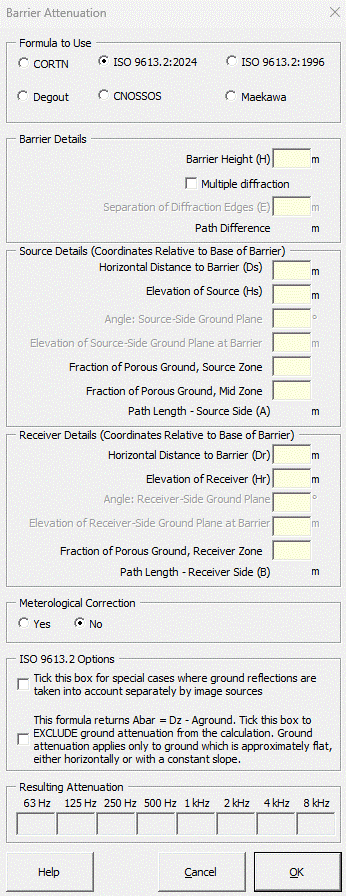### Strutt Help

Barrier Attenuation    1/1

Strutt|Environmental Noise|Barrier Attenuation provides a barrier attenuation calculation, using either the Maekawa equation, ISO9613-2 equation, Degout equation or the CORTN method, which is entered in the active row of the worksheet.

The CNOSSOS method of Barrier + Ground attenuation is also available and is discussed in detail here.The calculation is valid for the shadow or illuminated zone of the barrier and is limited to a maximum attenuation of (-) 25 dB at any frequency.

The Maekawa equation is implemented within the shadow zone as:

IL = -[5 + 20log_10(sqrt(2 pi N_F)/tanh(sqrt(2 pi N_F)))]

where,
N_F = 2(delta/lambda) is the Fresnel number

delta is the path difference, m
lambda is the wavelength of sound, m

Within the illuminated zone, a log-log curve fit is used to calculate the barrier loss based on the figure in the original Maekawa paper.

The CORTN method gives a single-number dB(A) value, which is placed in Column E of the active row. In the Barrier Attenuation user form, the attenuation is displayed in the octave band cells, but the final result is a broadband value only, and selecting the CORTN method will NOT insert spectral values into the active row. The CORTN method is implemented using the following equation:

IL = A_0 + A_1 x + A_2 x^2 + A_3 x^3 + ... + A_n x^n

where,
x = log(delta) and delta is the path difference, m
The coefficients A_n are:

 A0 A1 A2 A3 A4 A5 A6 A7 -15.4 -8.26 -2.787 -0.831 -0.198 0.1539 0.12248 0.02175

The ISO 9613-2 method includes an option to calculate the effect of meteorological conditions on the barrier attenuation, and is calculated using:

A_(b a r) = -10log_10(3 + 20/lambda C_3 delta K_(met))

Where,
lambda is the wavelength of sound at the frequency in question
delta is the path difference between the diffracted sound and direct sound, calculated using:

delta = d_(ss) + d_(sr) - d

C_3 is a coefficient for double diffraction; C_3 = 1 for single diffraction
K_(met) is a meteorological correction factor calculated using:

K_(met) = e^((-1/2000 sqrt(d_(ss)d_(sr)d)/(2 delta)))

d_(ss) is the distance from the source to the first diffraction edge, m
d_(sr) is the distance from the second diffraction edge to the reciever, m
d is the straight-line distance between source and receiver, m

The Degout formula is calculated as follows:

IL = {(0 " for " \ N_F < -0.25 ),(-6 + 12 sqrt(-N_F) \ " for " \ -0.25 <= N_F < 0 \ ),(-6 - 12 sqrt(N_F) \ " for " \ 0 <= N_F < 0.25 \ ),(-8 - 8 sqrt(N_F) \ " for " \ 0.25 <= N_F < 1.0 \ ),(-16 - 10log_10(N_F) \ " for " N_F > 1.0 \ ):}